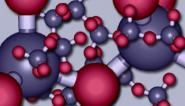The molecular world

Start this free course now. Just create an account and sign in. Enrol and complete the course for a free statement of participation or digital badge if available.

Free course

# 1.2.1 Isotopes

All atoms of the same element have identical atomic numbers, and are chemically similar, but they may not be identical in other ways. Figure 2f shows copper. All copper atoms have atomic number 29: all their nuclei contain 29 protons. But they also contain uncharged particles called neutrons. In natural copper, the atoms are of two kinds. One has 29 protons and 34 neutrons in the nucleus; the other has 29 protons and 36 neutrons (Figure 4).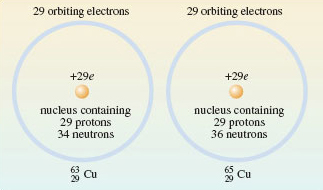Figure 4 The distribution of protons, neutrons and electrons in the atoms of the two isotopes of copper present in copper metal. In both cases, the atomic number is 29: there are 29 protons in the nucleus. This makes both types of atom, atoms of copper, but they differ in the number of neutrons contained in their nucleus

The two different kinds of atom are called isotopes of copper. The neutron has a mass very similar to that of the proton, so the two isotopes differ in mass. The sum of the numbers of neutrons and protons for a particular isotope is called the mass number.

## Question 3

What are the mass numbers of the two copper isotopes in Figure 4?

63 and 65 — that is, (29 + 34) and (29 + 36), respectively.

The two isotopes are written,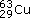and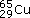where the mass number and atomic number precede the chemical symbol as a superscript and subscript, respectively (Figure 5).

The mass number of any isotope is equal to the relative atomic mass of its atom, rounded to the nearest whole number. The atoms of natural copper are about 70%and 30%. Thus, the relative atomic mass of natural copper (63.5) lies between 63 and 65, but closer to 63 because that is the relative atomic mass of the more abundant isotope. But although copper contains two different isotopes, each isotope has the same atomic number, and therefore a virtually identical chemistry.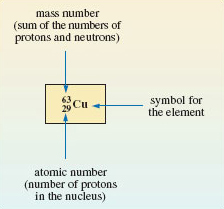Figure 5 A symbolism showing the number of neutrons, protons and electrons in the neutral atom of an isotope